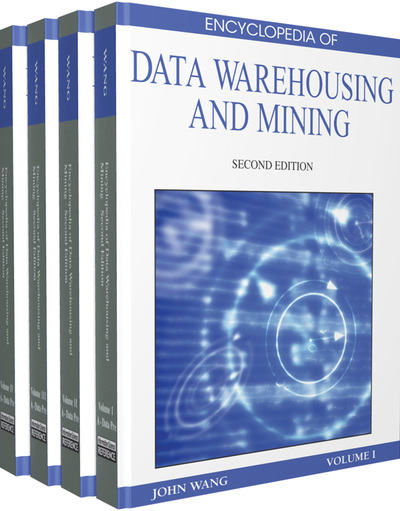# Multi-Instance Learning with MultiObjective Genetic Programming

Amelia Zafra (University of Cordoba, Spain)
DOI: 10.4018/978-1-60566-010-3.ch212
Available
\$37.50
No Current Special Offers

## Abstract

The multiple-instance problem is a difficult machine learning problem that appears in cases where knowledge about training examples is incomplete. In this problem, the teacher labels examples that are sets (also called bags) of instances. The teacher does not label whether an individual instance in a bag is positive or negative. The learning algorithm needs to generate a classifier that will correctly classify unseen examples (i.e., bags of instances). This learning framework is receiving growing attention in the machine learning community and since it was introduced by Dietterich, Lathrop, Lozano-Perez (1997), a wide range of tasks have been formulated as multi-instance problems. Among these tasks, we can cite content-based image retrieval (Chen, Bi, & Wang, 2006) and annotation (Qi and Han, 2007), text categorization (Andrews, Tsochantaridis, & Hofmann, 2002), web index page recommendation (Zhou, Jiang, & Li, 2005; Xue, Han, Jiang, & Zhou, 2007) and drug activity prediction (Dietterich et al., 1997; Zhou & Zhang, 2007). In this chapter we introduce MOG3P-MI, a multiobjective grammar guided genetic programming algorithm to handle multi-instance problems. In this algorithm, based on SPEA2, individuals represent classification rules which make it possible to determine if a bag is positive or negative. The quality of each individual is evaluated according to two quality indexes: sensitivity and specificity. Both these measures have been adapted to MIL circumstances. Computational experiments show that the MOG3P-MI is a robust algorithm for classification in different domains where achieves competitive results and obtain classifiers which contain simple rules which add comprehensibility and simplicity in the knowledge discovery process, being suitable method for solving MIL problems (Zafra & Ventura, 2007).
Chapter Preview
Top

## Background

In the middle of the 1990’s, Dietterich et al. (1997) described three Axis-Parallel Rectangle (abbreviated as APR) algorithms to solve the problem of classifying aromatic molecules according to whether or not they are “musky”. These methods attempted to search the appropriate axis-parallel rectangles constructed by their conjunction of features. Their best performing algorithm (iterated-discrim) started with a point in the feature space and grew a box with the goal of finding the smallest box covered at least one instance from each positive bag and no instances from any negative bag. The resulting box was then expanded (via a statistical technique) to get better results.

Following Dietterich et al.’s study, a wide variety of new methods of multi-instance learning has appeared. Auer (1997) tried to avoid some potentially hard computational problems that were required by the heuristics used in the iterated-discrim algorithm and presented a theoretical algorithm, MULTINST. With a new approach, Maron and Lozano-Perez (1998) proposed one of the most famous multi-instance learning algorithms, Diverse Density (DD), where the diverse density of a point, p, in the feature space was defined as a probabilistic measure which considered how many different positive bags had an instance near p, and how far the negative instances were from p. This algorithm was combined with the Expectation Maximization (EM) algorithm, appearing as EM-DD (Zhang & Goldman, 2001). Another study that extended the DD algorithm to maintain multilearning regression data sets was the EM-based multi-instance regression algorithm (Amar, Dooly, Goldman, & Zhang, 2001).

## Complete Chapter List

Search this Book:
Reset